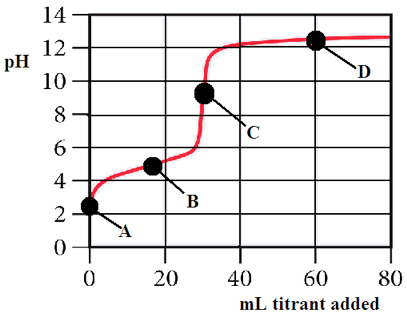# Problem: The following questions refer to the titration curve given below.  For each of the following questions circle the correct answer.           a) The titration curve shows the titration of  i. a strong acid with a strong base                             ii. a weak acid  with a strong base        iii. a strong base  with a strong acid                          iv. a weak base with a strong acid                                 b) Which point on the titration curve represents a region where a buffer solution has formed?            point A                          point B                          point C                          point Dc) Which point on the titration curve represents the equivalence point?            point A                          point B                          point C                          point Dd) Which of the following would be the best indicator to use in the titration?     i. erythrosin B  pKa = 2.9                      ii. methyl red pK   a = 5.4                                                iii. bromthymol blue pKa = 6.8             iv. o-cresonphthalein pK  a = 9.0

###### FREE Expert Solution
100% (315 ratings)
###### Problem Details

The following questions refer to the titration curve given below.  For each of the following questions circle the correct answer.

a) The titration curve shows the titration of

i. a strong acid with a strong base                             ii. a weak acid  with a strong base        iii. a strong base  with a strong acid                          iv. a weak base with a strong acid

b) Which point on the titration curve represents a region where a buffer solution has formed?

point A                          point B                          point C                          point D

c) Which point on the titration curve represents the equivalence point?

point A                          point B                          point C                          point D

d) Which of the following would be the best indicator to use in the titration?

i. erythrosin B  pK= 2.9                      ii. methyl red pK   = 5.4                                                iii. bromthymol blue pK= 6.8             iv. o-cresonphthalein pK  = 9.0What scientific concept do you need to know in order to solve this problem?

Our tutors have indicated that to solve this problem you will need to apply the Acid and Base Titration Curves concept. You can view video lessons to learn Acid and Base Titration Curves. Or if you need more Acid and Base Titration Curves practice, you can also practice Acid and Base Titration Curves practice problems.

What is the difficulty of this problem?

Our tutors rated the difficulty ofThe following questions refer to the titration curve given b...as medium difficulty.

How long does this problem take to solve?

Our expert Chemistry tutor, Jules took 5 minutes and 42 seconds to solve this problem. You can follow their steps in the video explanation above.

What professor is this problem relevant for?

Based on our data, we think this problem is relevant for Professor Wittenberg's class at LEHIGH.